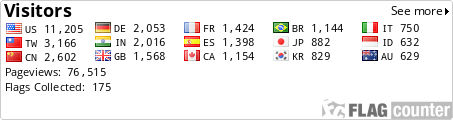About me | pbdR Tech | HPSC | Phyloclustering | R_note |

 R_note -- The Exploration of Statistical Software R (²Î­p³nÅé R ²`«×¾úÀI)
Reference
MS Windows

Batch jobs
Function
Data/MySQL
Plot
String/Parse

Remark Lines
Classes/S3
S4 Methods
Batch more
Environment

Good Coding
Fast Loop
LAM/MPI/Rmpi
Recursion

PHP Call R
Basic C
R Call Fortran/C
R Call GSL
C Call R API
C Call R Objects
Standalone

Make Packages
C Pointer
Debug

Section: Data/MySQL

• Read & Write
Function `read.table()` and `write.table()`.

 ``` read.table(file, header = FALSE, sep = "", quote = "\"'", dec = ".", row.names, col.names, as.is = FALSE, na.strings = "NA", colClasses = NA, nrows = -1, skip = 0, check.names = TRUE, fill = !blank.lines.skip, strip.white = FALSE, blank.lines.skip = TRUE, comment.char = "#") ``` ``` write.table(x, file = "", append = FALSE, quote = TRUE, sep = " ", eol = "\n", na = "NA", dec = ".", row.names = TRUE, col.names = TRUE, qmethod = c("escape", "double")) ```

• Load & Save
Function `read.table()` and `write.table()`.

 ``` load(file, envir = parent.frame()) ``` ``` save(..., list = character(0), file = stop("`file' must be specified"), ascii = FALSE, version = NULL, envir = parent.frame(), compress = FALSE) ```

For MS Windows:
R 1.6 and Mysql 3.x, DBI.zip or my mirror here, RMySQL.zip (my mirror here).
R 1.7 and Mysql 3.x or 4.x, DBI_0.1-5.zip or my mirror here, RMySQL_0.5-1.zip (my mirror here).
R 1.8 and Mysql 3.x or 4.x, DBI_0.1-8.zip or my mirror here, RMySQL_0.5-5.zip (my mirror here).
For Linux:
For R 1.7 and Mysql 3.x or 4.x, DBI_0.1-7.tar.gz or my mirror here, RMySQL_0.5-2.tar.gz (my mirror here).

• MySQL
Require packages `methods`, `DBI`, `RMySQL`.

 ``` # In Windows, require add the RMySQL.dll to PATH, like this # Sys.putenv(PATH = paste(Sys.getenv("PATH"), # "C:/PROGRA~1/R/rw1071/library/RMySQL/libs/", sep = ";")) library(methods) library(DBI) library(RMySQL) db <- NULL db\$info <- list(driver = "MySQL", user = "yourname", password = "yourpassword", host = "yourdbhost", port = "yourdbport") db\$drv <- dbDriver(db\$info\$driver) db\$con <- dbConnect(db\$drv, user = db\$info\$user, password = db\$info\$password, host = db\$info\$host, port = db\$info\$port, dbname = "whatyouwant") tb <- NULL tb\$get <- dbGetQuery(db\$con, paste("select * from table where field = \"", value, sep = "")) dbDisconnect(db\$con) dbUnloadDriver(db\$drv) ```

• More
Another database, ODBC, PostgreSQL, Oracle, ....
See R & DBMSs Page

 [ Go to top ]

 Maintained: Wei-Chen Chen E-Mail: wccsnow @ gmail.com Last Revised: Dec 12 2016, 09:44 (CST Taipei, Taiwan) Created: Oct 06 2003Best Resolution IE6.0 1280x1024 small font